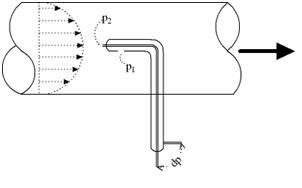# Pitot Tube (Henri Pitot (1695–1771))Pitot Tube
INTRODUCTION
Instrument for measuring the velocity (speed) of a flowing fluid. Invented by Henri Pitot (1695–1771), it consists of a tube with a short, right-angled bend, which is placed vertically in a moving fluid with the mouth of the bent part directed upstream; the pressure, measured with an attached device, depends on the fluid flow and can be used to calculate the velocity. Pitot tubes are used to measure airspeed in wind tunnels and aboard aircraft in flight; they are also used to measure the flow of liquids.A Pitot-static tube can measure the fluid flow velocity by converting the kinetic energy in the fluid flow into potential energy.

EXPLANATION
The principle is based on the Bernoulli Equation where each term can be interpreted as a form of pressure,

p + 1/2 ρ v2 + γ h = constant along a streamline (1)

where

p = static pressure (relative to the moving fluid) (Pa)

ρ = density (kg/m3)

γ = specific weight (kN/m3)

v = flow velocity (m/s)

g = acceleration of gravity (m/s2)

h = elevation height (m)

Each term of this equation has the dimension force per unit area - N/m2 or in imperial units psi, lb/ft2.
Static Pressure

The first term - p - is the static pressure. It is static relative to the moving fluid and can be measured through an flat opening in parallel to the flow.
Dynamic Pressure

The second term - 1/2 ρ v2 - is called the dynamic pressure.
Hydrostatic Pressure

The third term - γ h - is called the hydrostatic pressure. It represent the pressure due to change in elevation.
Stagnation Pressure

Since the Bernoulli Equation states that the energy along the streamline is constant, (1) can be modified to

p1 + 1/2 ρ v12 + γ h1

= p2 + 1/2 ρ v22 + γ h2

= constant along the streamline (2)

where

suffix 1 is a point in the free flow upstream

suffix 2 is the stagnation point where the velocity in the flow is zero
Flow Velocity

In a measuring point we regard the hydrostatic pressure as a constant, h1 = h2 and this part can be eliminated. Since v2 is zero, (2) can be modified to:

p1 + 1/2 ρ v12 = p2 (3)

or

v1 = [ 2 (p2 - p1) / ρ ] 1/2 (4)

where

p2 - p1 = dp (differential pressure)

With (4) it's possible to calculate the flow velocity in point 1 - the free flow upstream - if we know the differential pressure difference dp = p2 - p1 and the density of the fluid.

The pitot tube is a simple and convenient instrument to measure the difference between static, dynamic and total pressure (or head).

[blogger]

Engineeersdaily

Name

Email *

Message *